# LOG#026. Boosts, rapidity, HEP.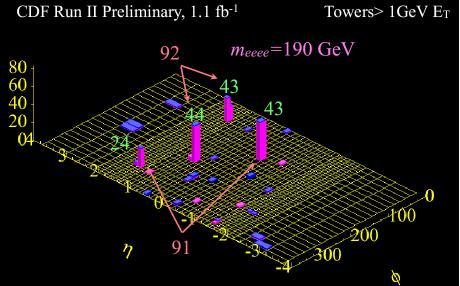In euclidean two dimensional space, rotations are easy to understand in terms of matrices and trigonometric functions. A plane rotation is given by: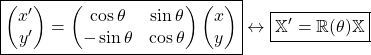where the rotation angle is, and it is parametrized by.

Interestingly, in minkovskian two dimensional spacetime, the analogue does exist and it is written in terms of matrices and hyperbolic trigonometric functions. A “plane” rotation in spacetime is given by:Here,is the so-called hiperbolic rotation angle, pseudorotation, or more commonly, the rapidity of the Lorentz boost in 2d spacetime. It shows that rapidity are a very useful parameter for calculations in Special Relativity. Indeed, it is easy to check that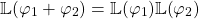So, at least in the 2d spacetime case, rapidities are “additive” in the written sense.

Firstly, we are going to guess the relationship between rapidity and velocity in a single lorentzian spacetime boost. From the above equation we get: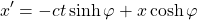Multiplying the first equation byand the second one by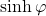, we add the resulting equation to obtain: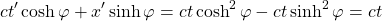that is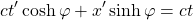From this equation (or the boxed equations), we see thatcorresponds to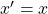and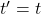. Setting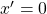, we deduce thatand thusor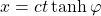.

Since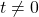, and the pseudorotation seems to have a “pseudovelocity” equals to, the rapidity it is then defined through the equation: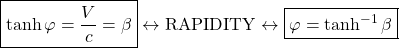If we remember what we have learned in our previous mathematical survey, that is,We setin order to get the next alternative expression for the rapidity: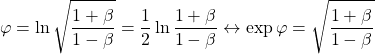In experimental particle physics, in general 3+1 spacetime, the rapidity definition is extended as follows. Writing, from the previous equations above,and using these two last equations, we can also write momenergy components using rapidity in the same fashion. Suppose that for some particle(object), its  mass is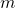, its energy is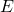, and its (relativistic) momentum is. Then: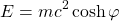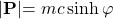From these equations, it is trivial to guess:This is the completely general definition of rapidity used in High Energy Physics (HEP), with a further detail. In HEP, physicists used to select the direction of momentum in the same direction that the collision beam particles! Suppose we select some orientation, e.g.the z-axis. Then,and rapidity is defined in that beam direction as: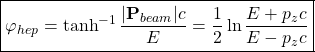In 2d spacetime, rapidities add nonlinearly according to the celebrated relativistic addition rule:Indeed, Lorentz transformations do commute in 2d spacetime since we boost in a same direction x, we get: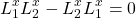with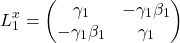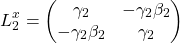This commutativity is lost when we go to higher dimensions. Indeed, in spacetime with more than one spatial direction that result is not true in general. If we build a Lorentz transformation with two boosts in different directions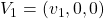and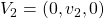, the Lorentz matrices are ( remark for experts: we leave one direction in space untouched, so we get 3×3 matrices):and it is easily checked thatFinally, there is other related quantity to rapidity that even experimentalists do prefer to rapidity. It is called: PSEUDORAPIDITY!

Pseudorapidity, often denoted by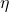describes the angle of a particle relative to the beam axis. Mathematically speaking is: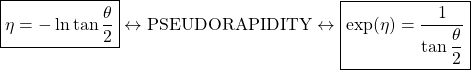whereis the angle between the particle momentumand the beam axis. The above relation can be inverted to provide: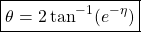The pseudorapidity in terms of the momentum is given by: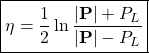Note that, unlike rapidity, pseudorapidity depends only on the polar angle of its trajectory, and not on the energy of the particle.

In hadron collider physics,  and other colliders as well, the rapidity (or pseudorapidity) is preferred over the polar angle because, loosely speaking, particle production is constant as a function of rapidity. One speaks of the “forward” direction in a collider experiment, which refers to regions of the detector that are close to the beam axis, at high pseudorapidity.

The rapidity as a function of pseudorapidity is provided by the following formula: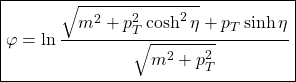where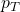is the momentum transverse to the direction of motion and m is the invariant mass of the particle.

Remark: The difference in the rapidity of two particles is independent of the Lorentz boosts along the beam axis.

Colliders measure physical momenta in terms of transverse momentuminstead of the momentum in the direction of the beam axis (longitudinal momentum)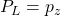, the polar angle in the transverse plane (genarally denoted by) and pseudorapidity. To obtain cartesian momenta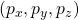(with the z-axis defined as the beam axis), the following transformations are used: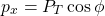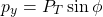Thus, we get the also useful relationship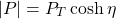This quantity is an observable in the collision of particles, and it can be measured as the main image of this post shows.

This site uses Akismet to reduce spam. Learn how your comment data is processed.